# 图像处理为什么不用dst

## [Python图像处理] 七.图像阈值化处理及算法对比

2018-10-30 22:19:36 Eastmount 阅读数 8400
• ###### 再论图像分割

掌握图像去噪滤波方法及各自特点； 掌握常用的图像边缘检测算子，及Canny算子基本原理 掌握灰度阈值化及大津算法； 掌握常见的图像特征描述方式 了解局部阈值分割、区域生长、分水岭算法及基于轮廓的阈值分割； 通过...

2537人学习 CSDN就业班
免费试看

PS：请求帮忙点个Star，哈哈，第一次使用Github，以后会分享更多代码，一起加油。

1.阈值化
2.二进制阈值化
3.反二进制阈值化
4.截断阈值化
5.反阈值化为0
6.阈值化为0

PS：文章参考自己以前系列图像处理文章及OpenCV库函数，同时部分参考网易云视频，推荐大家去学习。同时，本篇文章涉及到《计算机图形学》基础知识，请大家下来补充。

PSS：2019年1~2月作者参加了CSDN2018年博客评选，希望您能投出宝贵的一票。我是59号，Eastmount，杨秀璋。投票地址：https://bss.csdn.net/m/topic/blog_star2018/index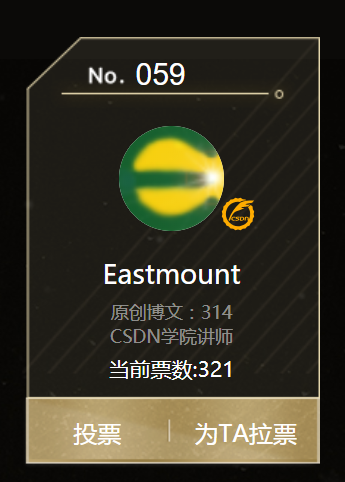# 一. 阈值化

（注：该部分参考作者的论文《基于苗族服饰的图像锐化和边缘提取技术研究》）

$\begin{cases} Y=0，gray=T\\ \end{cases}$

Python OpenCV中提供了阈值函数threshold()实现二值化处理，其公式及参数如下图所示：
retval, dst = cv2.threshold(src, thresh, maxval, type)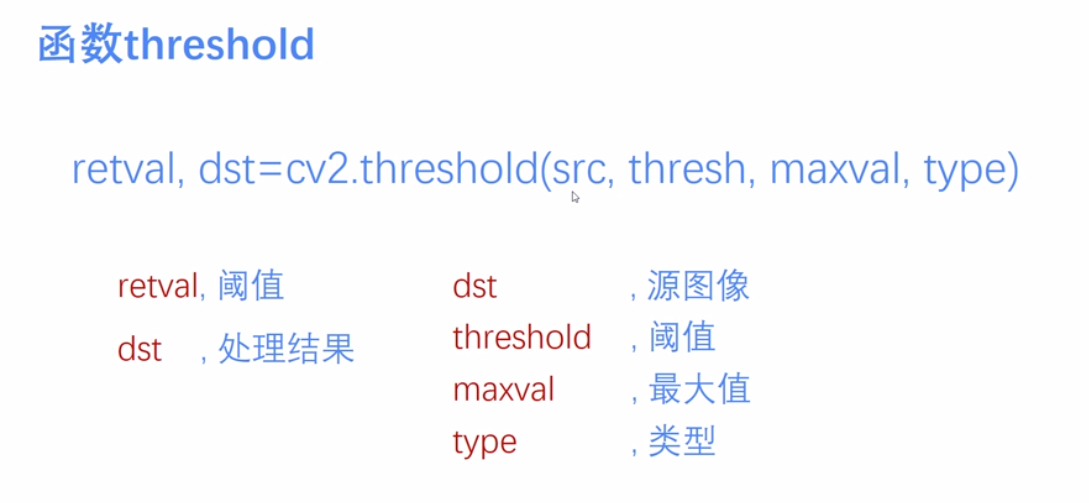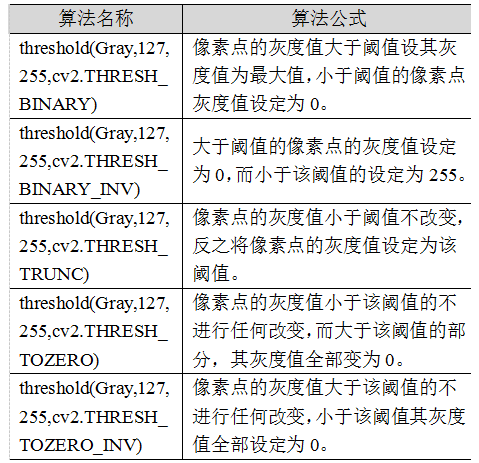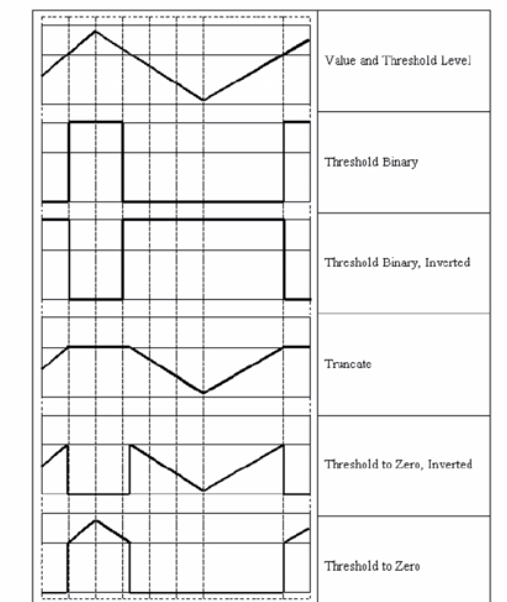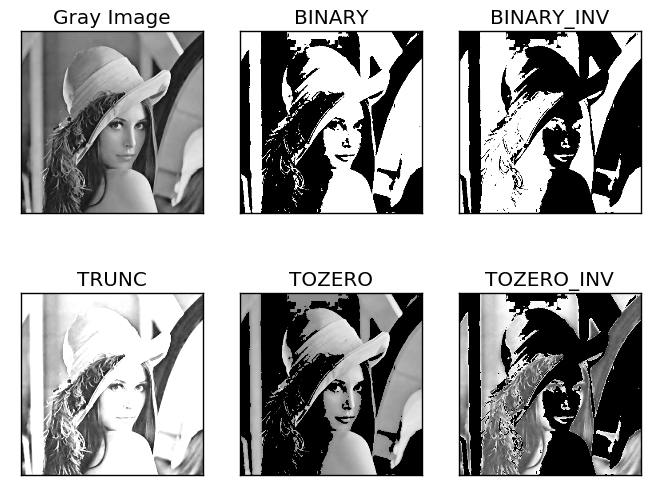# 二. 二进制阈值化

$dst(x,y) = \begin{cases} maxVal， if src(x,y)>thresh\\ 0，otherwise\\ \end{cases}$
(1) 大于等于127的像素点的灰度值设定为最大值（如8位灰度值最大为255）
(2) 灰度值小于127的像素点的灰度值设定为0

#encoding:utf-8
import cv2
import numpy as np

#读取图片

#灰度图像处理
GrayImage = cv2.cvtColor(src,cv2.COLOR_BGR2GRAY)

#二进制阈值化处理
r, b = cv2.threshold(GrayImage, 127, 255, cv2.THRESH_BINARY)
print r

#显示图像
cv2.imshow("src", src)
cv2.imshow("result", b)

#等待显示
cv2.waitKey(0)
cv2.destroyAllWindows()# 三. 反二进制阈值化

$dst(x,y) = \begin{cases} 0， if src(x,y)>thresh\\ maxVal，otherwise\\ \end{cases}$
(1) 大于127的像素点的灰度值设定为0（以8位灰度图为例）
(2) 小于该阈值的灰度值设定为255

#encoding:utf-8
import cv2
import numpy as np

#读取图片

#灰度图像处理
GrayImage = cv2.cvtColor(src,cv2.COLOR_BGR2GRAY)

#反二进制阈值化处理
r, b = cv2.threshold(GrayImage, 127, 255, cv2.THRESH_BINARY_INV)
print r

#显示图像
cv2.imshow("src", src)
cv2.imshow("result", b)

#等待显示
cv2.waitKey(0)
cv2.destroyAllWindows()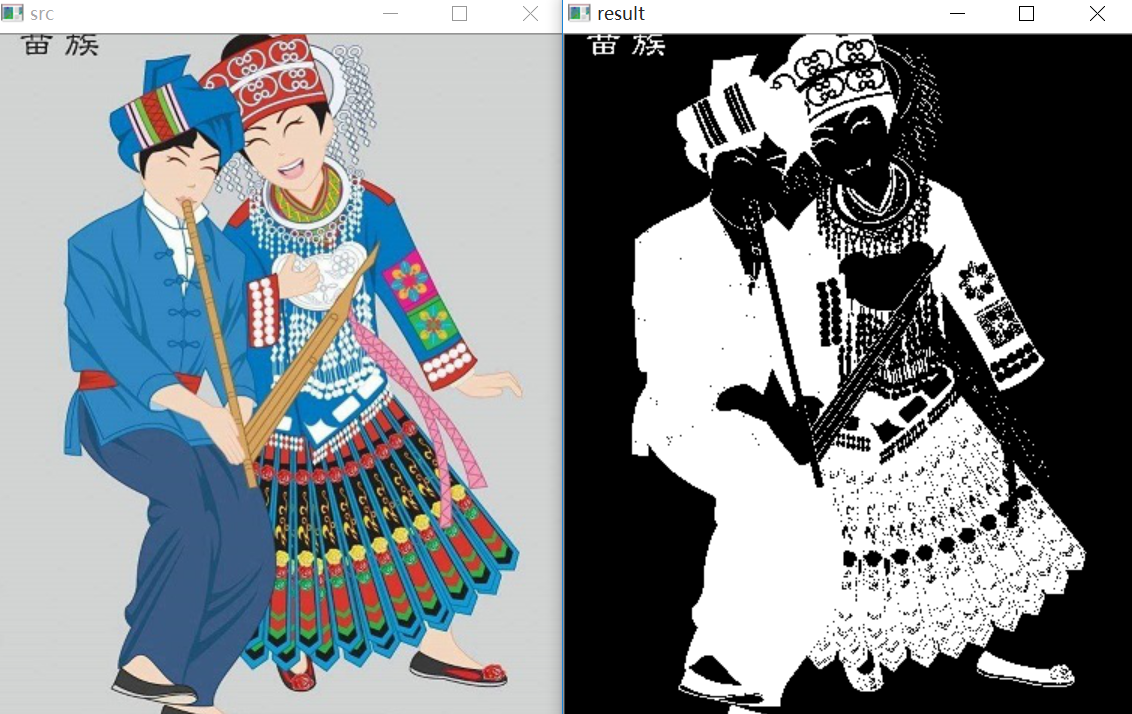# 四. 截断阈值化

$dst(x,y) = \begin{cases} threshold， if src(x,y)>thresh\\ src(x,y)，otherwise\\ \end{cases}$
(1) 大于等于127的像素点的灰度值设定为该阈值127
(2) 小于该阈值的灰度值不改变

#encoding:utf-8
import cv2
import numpy as np

#读取图片

#灰度图像处理
GrayImage = cv2.cvtColor(src,cv2.COLOR_BGR2GRAY)

#截断阈值化处理
r, b = cv2.threshold(GrayImage, 127, 255, cv2.THRESH_TRUNC)
print r

#显示图像
cv2.imshow("src", src)
cv2.imshow("result", b)

#等待显示
cv2.waitKey(0)
cv2.destroyAllWindows()# 五. 反阈值化为0

$dst(x,y) = \begin{cases} 0， if src(x,y)>thresh\\ src(x,y)，otherwise\\ \end{cases}$
(1) 大于等于阈值127的像素点变为0
(2) 小于该阈值的像素点值保持不变

#encoding:utf-8
import cv2
import numpy as np

#读取图片

#灰度图像处理
GrayImage = cv2.cvtColor(src,cv2.COLOR_BGR2GRAY)

#反阈值化为0处理
r, b = cv2.threshold(GrayImage, 127, 255, cv2.THRESH_TOZERO_INV)
print r

#显示图像
cv2.imshow("src", src)
cv2.imshow("result", b)

#等待显示
cv2.waitKey(0)
cv2.destroyAllWindows()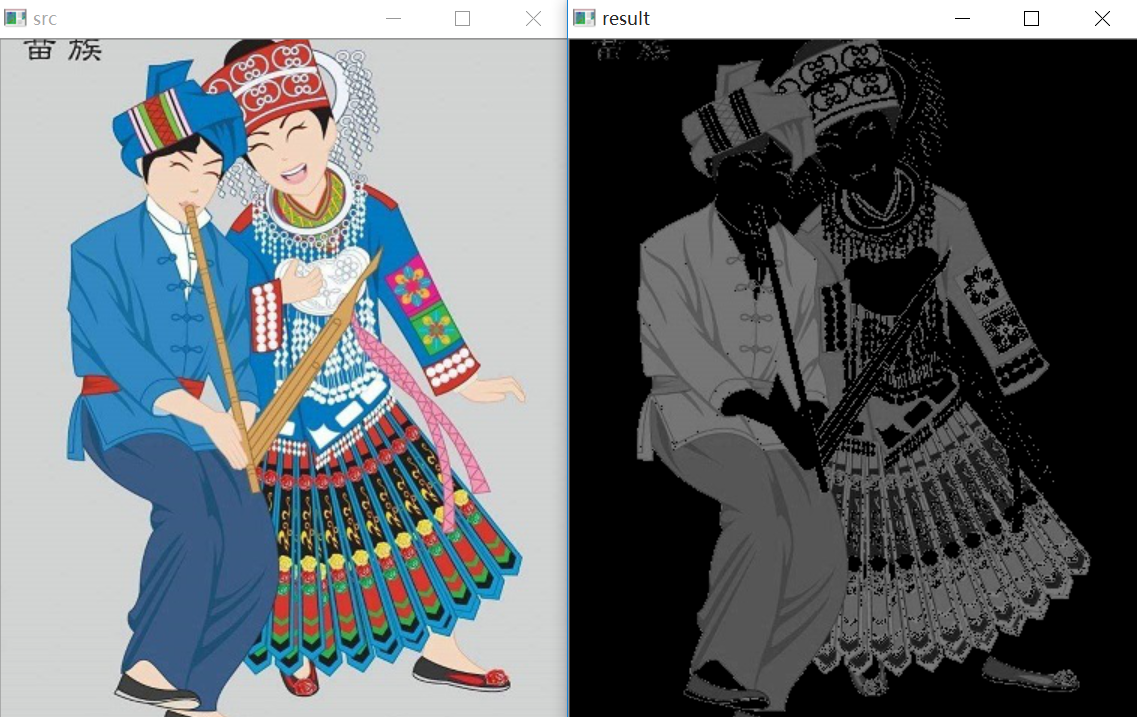# 六. 阈值化为0

$dst(x,y) = \begin{cases} src(x,y)， if src(x,y)>thresh\\ 0，otherwise\\ \end{cases}$
(1) 大于等于阈值127的像素点，值保持不变
(2) 小于该阈值的像素点值设置为0

#encoding:utf-8
import cv2
import numpy as np

#读取图片

#灰度图像处理
GrayImage = cv2.cvtColor(src,cv2.COLOR_BGR2GRAY)

#阈值化为0处理
r, b = cv2.threshold(GrayImage, 127, 255, cv2.THRESH_TOZERO)
print r

#显示图像
cv2.imshow("src", src)
cv2.imshow("result", b)

#等待显示
cv2.waitKey(0)
cv2.destroyAllWindows()#encoding:utf-8
import cv2
import numpy as np
import matplotlib.pyplot as plt

#读取图像
lenna_img = cv2.cvtColor(img,cv2.COLOR_BGR2RGB)
GrayImage=cv2.cvtColor(img,cv2.COLOR_BGR2GRAY)

#阈值化处理
ret,thresh1=cv2.threshold(GrayImage,127,255,cv2.THRESH_BINARY)
ret,thresh2=cv2.threshold(GrayImage,127,255,cv2.THRESH_BINARY_INV)
ret,thresh3=cv2.threshold(GrayImage,127,255,cv2.THRESH_TRUNC)
ret,thresh4=cv2.threshold(GrayImage,127,255,cv2.THRESH_TOZERO)
ret,thresh5=cv2.threshold(GrayImage,127,255,cv2.THRESH_TOZERO_INV)

#显示结果
titles = ['Gray Image','BINARY','BINARY_INV','TRUNC','TOZERO','TOZERO_INV']
images = [GrayImage, thresh1, thresh2, thresh3, thresh4, thresh5]
for i in xrange(6):
plt.subplot(2,3,i+1),plt.imshow(images[i],'gray')
plt.title(titles[i])
plt.xticks([]),plt.yticks([])
plt.show()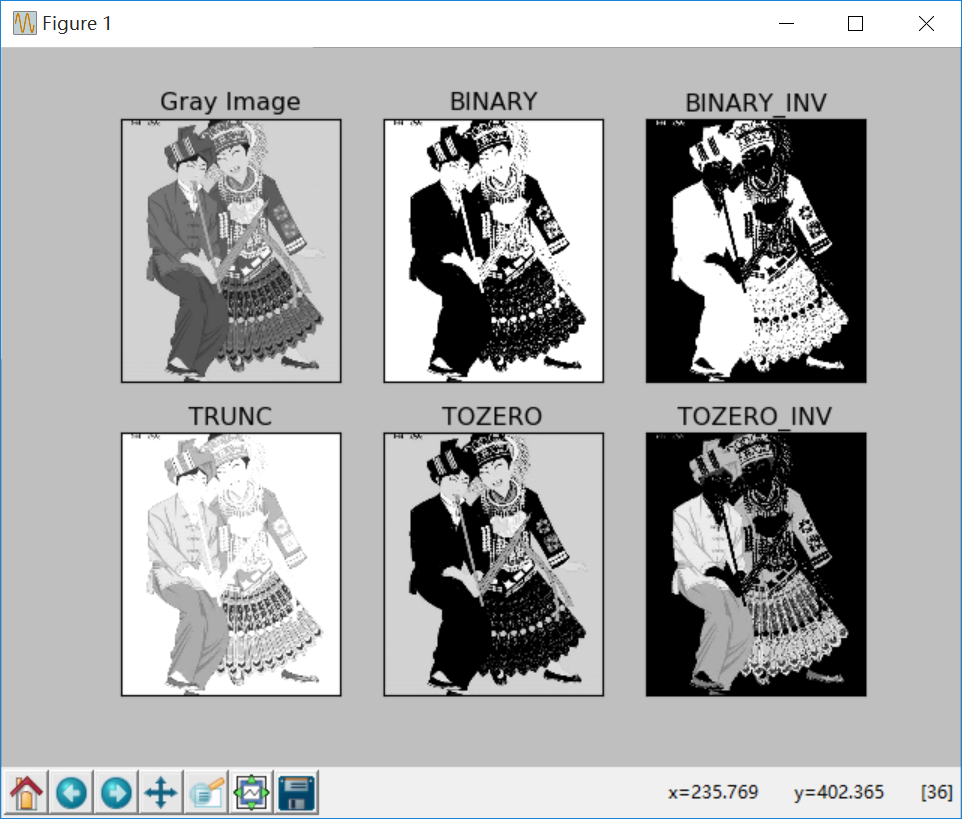（By：Eastmount 2018-10-30 晚上10点 https://blog.csdn.net/Eastmount/）

## 图像处理中的傅里叶变换

2017-04-15 19:34:01 m0_37264397 阅读数 61094
• ###### 再论图像分割

掌握图像去噪滤波方法及各自特点； 掌握常用的图像边缘检测算子，及Canny算子基本原理 掌握灰度阈值化及大津算法； 掌握常见的图像特征描述方式 了解局部阈值分割、区域生长、分水岭算法及基于轮廓的阈值分割； 通过...

2537人学习 CSDN就业班
免费试看

## 图形图像处理－之－高质量的快速的图像缩放 中篇 二次线性插值和三次卷积插值

2006-12-21 21:02:00 housisong 阅读数 30504
• ###### 再论图像分割

掌握图像去噪滤波方法及各自特点； 掌握常用的图像边缘检测算子，及Canny算子基本原理 掌握灰度阈值化及大津算法； 掌握常见的图像特征描述方式 了解局部阈值分割、区域生长、分水岭算法及基于轮廓的阈值分割； 通过...

2537人学习 CSDN就业班
免费试看

图形图像处理－之－高质量的快速的图像缩放 中篇 二次线性插值和三次卷积插值
HouSisong@GMail.com   2006255.12.13

(2015.08.15  PicZoom_ftBilinear_Common更精确的边界公式推导)

(2009.03.07  可以到这里下载缩放算法的完整的可以编译的项目源代码:  http://blog.csdn.net/housisong/archive/2009/03/07/3967270.aspx  )

(2007.11.12 替换了二次线性插值的实现(以前偷懒使用了一个近似公式），改进后在图片边缘的插值效果更好(包括三次卷积插值的边界也更精确)；
(2007.09.14 修正三次卷积的MMX版本中表的精度太低(7bit),造成卷积结果误差较大的问题,该版本提高了插值质量,并且速度加快12-25%)
(2007.09.07 PicZoom_ThreeOrder2和PicZoom_ThreeOrder_MMX在缩放的图片宽或高

yrIntFloat_16的定义改成long xrIntFloat_16,yrIntFloat_16就可以了)
(2007.07.02 ThreeOrder2_Fast一点小的改进,加快14%)
(2007.06.18 优化PicZoom_BilInear_MMX的实现(由138.5fps提高到147.9fps),
并添加更快的两路展开的实现版本BilInear_MMX_expand2函数；
补充新的SSE2的实现PicZoom_BilInear_SSE2函数)
(2007.06.06 更新测试数据,编译器由vc6改为vc2005,CPU由赛扬2G改为AMD64x2 4200+(2.1G) )
(2007.03.06 更新)

tag:图像缩放,速度优化,定点数优化,近邻取样插值,二次线性插值,三次线性插值,
MipMap链,三次卷积插值,MMX,SSE,SSE2,CPU缓存优化

上篇 近邻取样插值和其速度优化
中篇 二次线性插值和三次卷积插值
下篇 三次线性插值和MipMap链

补充 使用SSE2优化

为了便于讨论，这里只处理32bit的ARGB颜色；
代码使用C++;涉及到汇编优化的时候假定为x86平台;使用的编译器为vc2005;
为了代码的可读性,没有加入异常处理代码;
测试使用的CPU为AMD64x2 4200+(2.37G)  和 Intel Core2 4400(2.00G);

只测试内存数据到内存数据的缩放
测试图片都是800*600缩放到1024*768; fps表示每秒钟的帧数,值越大表示函数越快

A:近邻取样插值、二次线性插值、三次卷积插值 缩放效果对比原图         近邻取样缩放到0.6倍     近邻取样缩放到1.6倍二次线性插值缩放到0.6倍   二次线性插值缩放到1.6倍三次卷积插值缩放到0.6倍   三次卷积插值缩放到1.6倍原图 近邻取样缩放到8倍 二次线性插值缩放到8倍 三次卷积插值缩放到8倍 二次线性插值(近似公式)

近邻取样插值缩放简单、速度快，但很多时候缩放出的图片质量比较差(特别是对于人物、景色等),

B: 首先定义图像数据结构:

#define asm __asm

typedef unsigned
char TUInt8; // [0..255]
struct TARGB32      //32 bit color
{
TUInt8  b,g,r,a;
//a is alpha
};

struct TPicRegion  //一块颜色数据区的描述，便于参数传递
{
TARGB32
*    pdata;         //颜色数据首地址
long        byte_width;    //一行数据的物理宽度(字节宽度)；

//abs(byte_width)有可能大于等于width*sizeof(TARGB32);
long        width;         //像素宽度
long        height;        //像素高度
};

//那么访问一个点的函数可以写为：
inline TARGB32& Pixels(const TPicRegion& pic,const long x,const long y)
{

return ( (TARGB32*)((TUInt8*)pic.pdata+pic.byte_width*y) )[x];
}

C: 二次线性插值缩放原理和公式图示:缩放后图片                 原图片
(宽DW,高DH)              (宽SW,高SH)

缩放映射原理:
(Sx-0)/(SW-0)=(Dx-0)/(DW-0)   (Sy-0)/(SH-0)=(Dy-0)/(DH-0)
=>   Sx=Dx*SW/DW                    Sy=Dy*SH/DH

聚焦看看(Sx,Sy)坐标点(Sx,Sy为浮点数)附近的情况；对于近邻取样插值的缩放算法，直接取Color0颜色作为缩放后点的颜色；

( u=Sx-floor(Sx); v=Sy-floor(Sy); 说明:floor函数的返回值为小于等于参数的最大整数 )
二次线性插值公式为:
tmpColor0=Color0*(1-u) + Color2*u;
tmpColor1=Color1*(1-u) + Color3*u;
DstColor =tmpColor0*(1-v) + tmpColor2*v;

展开公式为:
pm0=(1-u)*(1-v);
pm1=v*(1-u);
pm2=u*(1-v);
pm3=u*v;
则颜色混合公式为:
DstColor = Color0*pm0 + Color1*pm1 + Color2*pm2 + Color3*pm3;二次线性插值函数图示

=>   Sx=(Dx+0.5)*SW/DW-0.5; Sy=(Dy+0.5)*SH/DH-0.5;

//访问一个点的函数，(x,y)坐标可能超出图片边界； //边界处理模式:边界饱和
inline TARGB32 Pixels_Bound(const TPicRegion& pic,long x,long y)
{

//assert((pic.width>0)&&(pic.height>0));
bool IsInPic=true;

if (x<0) {x=0; IsInPic=false; } else if (x>=pic.width ) {x=pic.width -1; IsInPic=false; }

if (y<0) {y=0; IsInPic=false; } else if (y>=pic.height) {y=pic.height-1; IsInPic=false; }
TARGB32 result
=Pixels(pic,x,y);

if (!IsInPic) result.a=0;

return result;
}

D: 二次线性插值缩放算法的一个参考实现：PicZoom_BilInear0
该函数并没有做什么优化，只是一个简单的浮点实现版本；

inline void Bilinear0(const TPicRegion& pic,float fx,float fy,TARGB32* result)
{

long x=(long)fx; if (x>fx) --x; //x=floor(fx);
long y=(long)fy; if (y>fy) --y; //y=floor(fy);

TARGB32 Color0
=Pixels_Bound(pic,x,y);
TARGB32 Color2
=Pixels_Bound(pic,x+1,y);
TARGB32 Color1
=Pixels_Bound(pic,x,y+1);
TARGB32 Color3
=Pixels_Bound(pic,x+1,y+1);

float u=fx-x;

float v=fy-y;

float pm3=u*v;

float pm2=u*(1-v);

float pm1=v*(1-u);

float pm0=(1-u)*(1-v);

result
->a=(pm0*Color0.a+pm1*Color1.a+pm2*Color2.a+pm3*Color3.a);
result
->r=(pm0*Color0.r+pm1*Color1.r+pm2*Color2.r+pm3*Color3.r);
result
->g=(pm0*Color0.g+pm1*Color1.g+pm2*Color2.g+pm3*Color3.g);
result
->b=(pm0*Color0.b+pm1*Color1.b+pm2*Color2.b+pm3*Color3.b);
}

void PicZoom_Bilinear0(const TPicRegion& Dst,const TPicRegion& Src)
{

if (  (0==Dst.width)||(0==Dst.height)

||(0==Src.width)||(0==Src.height)) return;

unsigned
long dst_width=Dst.width;
TARGB32
* pDstLine=Dst.pdata;

for (unsigned long y=0;y<Dst.height;++y)
{

float srcy=(y+0.4999999)*Src.height/Dst.height-0.5;

for (unsigned long x=0;x<dst_width;++x)
{

float srcx=(x+0.4999999)*Src.width/Dst.width-0.5;
Bilinear0(Src,srcx,srcy,
&pDstLine[x]);
}
((TUInt8
*&)pDstLine)+=Dst.byte_width;
}
}

////////////////////////////////////////////////////////////////////////////////
//速度测试:
//==============================================================================
// PicZoom_BilInear0      8.3 fps
////////////////////////////////////////////////////////////////////////////////

E: 把PicZoom_BilInear0的浮点计算改写为定点数实现：PicZoom_BilInear1

inline void Bilinear1(const TPicRegion& pic,const long x_16,const long y_16,TARGB32* result)
{

long x=x_16>>16;

long y=y_16>>16;
TARGB32 Color0
=Pixels_Bound(pic,x,y);
TARGB32 Color2
=Pixels_Bound(pic,x+1,y);
TARGB32 Color1
=Pixels_Bound(pic,x,y+1);
TARGB32 Color3
=Pixels_Bound(pic,x+1,y+1);

unsigned
long u_8=(x_16 & 0xFFFF)>>8;
unsigned
long v_8=(y_16 & 0xFFFF)>>8;
unsigned
long pm3_16=(u_8*v_8);
unsigned
long pm2_16=(u_8*(unsigned long)(256-v_8));
unsigned
long pm1_16=(v_8*(unsigned long)(256-u_8));
unsigned
long pm0_16=((256-u_8)*(256-v_8));

result
->a=((pm0_16*Color0.a+pm1_16*Color1.a+pm2_16*Color2.a+pm3_16*Color3.a)>>16);
result
->r=((pm0_16*Color0.r+pm1_16*Color1.r+pm2_16*Color2.r+pm3_16*Color3.r)>>16);
result
->g=((pm0_16*Color0.g+pm1_16*Color1.g+pm2_16*Color2.g+pm3_16*Color3.g)>>16);
result
->b=((pm0_16*Color0.b+pm1_16*Color1.b+pm2_16*Color2.b+pm3_16*Color3.b)>>16);
}

void PicZoom_Bilinear1(const TPicRegion& Dst,const TPicRegion& Src)
{

if (  (0==Dst.width)||(0==Dst.height)

||(0==Src.width)||(0==Src.height)) return;

long xrIntFloat_16=((Src.width)<<16)/Dst.width

long yrIntFloat_16=((Src.height)<<16)/Dst.height;

const long csDErrorX=-(1<<15)+(xrIntFloat_16>>1);

const long csDErrorY=-(1<<15)+(yrIntFloat_16>>1);

unsigned
long dst_width=Dst.width;

TARGB32
* pDstLine=Dst.pdata;

long srcy_16=csDErrorY;

long y;

for (y=0;y<Dst.height;++y)
{

long srcx_16=csDErrorX;

for (unsigned long x=0;x<dst_width;++x)
{
Bilinear1(Src,srcx_16,srcy_16,
&pDstLine[x]); //border
srcx_16+=xrIntFloat_16;
}
srcy_16
+=yrIntFloat_16;
((TUInt8
*&)pDstLine)+=Dst.byte_width;
}

////////////////////////////////////////////////////////////////////////////////
//速度测试:
//==============================================================================
// PicZoom_BilInear1     17.7 fps
////////////////////////////////////////////////////////////////////////////////

F: 二次线性插值需要考略边界访问超界的问题，我们可以将边界区域和内部区域分开处理，这样就可以优化内部的插值实现函数了：比如不需要判断访问超界、减少颜色数据复制、减少一些不必要的重复坐标计算等等

inline void Bilinear2_Fast(TARGB32* PColor0,TARGB32* PColor1,unsigned long u_8,unsigned long v_8,TARGB32* result)
{
unsigned
long pm3_16=u_8*v_8;
unsigned
long pm2_16=(u_8<<8)-pm3_16;
unsigned
long pm1_16=(v_8<<8)-pm3_16;
unsigned
long pm0_16=(1<<16)-pm1_16-pm2_16-pm3_16;

result
->a=((pm0_16*PColor0.a+pm2_16*PColor0.a+pm1_16*PColor1.a+pm3_16*PColor1.a)>>16);
result
->r=((pm0_16*PColor0.r+pm2_16*PColor0.r+pm1_16*PColor1.r+pm3_16*PColor1.r)>>16);
result
->g=((pm0_16*PColor0.g+pm2_16*PColor0.g+pm1_16*PColor1.g+pm3_16*PColor1.g)>>16);
result
->b=((pm0_16*PColor0.b+pm2_16*PColor0.b+pm1_16*PColor1.b+pm3_16*PColor1.b)>>16);
}

inline
void Bilinear2_Border(const TPicRegion& pic,const long x_16,const long y_16,TARGB32* result)
{

long x=(x_16>>16);

long y=(y_16>>16);
unsigned
long u_16=((unsigned short)(x_16));
unsigned
long v_16=((unsigned short)(y_16));

TARGB32 pixel[
4];
pixel[
0]=Pixels_Bound(pic,x,y);
pixel[
1]=Pixels_Bound(pic,x+1,y);
pixel[
2]=Pixels_Bound(pic,x,y+1);
pixel[
3]=Pixels_Bound(pic,x+1,y+1);

Bilinear2_Fast(
&pixel,&pixel,u_16>>8,v_16>>8,result);
}

void PicZoom_Bilinear2(const TPicRegion& Dst,const TPicRegion& Src)
{

if (  (0==Dst.width)||(0==Dst.height)

||(0==Src.width)||(0==Src.height)) return;

long xrIntFloat_16=((Src.width)<<16)/Dst.width

long yrIntFloat_16=((Src.height)<<16)/Dst.height;

const long csDErrorX=-(1<<15)+(xrIntFloat_16>>1);

const long csDErrorY=-(1<<15)+(yrIntFloat_16>>1);

unsigned
long dst_width=Dst.width;

//计算出需要特殊处理的边界
long border_y0=-csDErrorY/yrIntFloat_16+1;              //y0+y*yr>=0; y0=csDErrorY => y>=-csDErrorY/yr
if (border_y0>=Dst.height) border_y0=Dst.height;

long border_x0=-csDErrorX/xrIntFloat_16+1;

if (border_x0>=Dst.width ) border_x0=Dst.width;

long border_y1=(((Src.height-2)<<16)-csDErrorY)/yrIntFloat_16+1//y0+y*yr<=(height-2) => y<=(height-2-csDErrorY)/yr
if (border_y1<border_y0) border_y1=border_y0;

long border_x1=(((Src.width-2)<<16)-csDErrorX)/xrIntFloat_16+1

if (border_x1<border_x0) border_x1=border_x0;

TARGB32
* pDstLine=Dst.pdata;

long Src_byte_width=Src.byte_width;

long srcy_16=csDErrorY;

long y;

for (y=0;y<border_y0;++y)
{

long srcx_16=csDErrorX;

for (unsigned long x=0;x<dst_width;++x)
{
Bilinear2_Border(Src,srcx_16,srcy_16,
&pDstLine[x]); //border
srcx_16+=xrIntFloat_16;
}
srcy_16
+=yrIntFloat_16;
((TUInt8
*&)pDstLine)+=Dst.byte_width;
}

for (y=border_y0;y<border_y1;++y)
{

long srcx_16=csDErrorX;

long x;

for (x=0;x<border_x0;++x)
{
Bilinear2_Border(Src,srcx_16,srcy_16,
&pDstLine[x]);//border
srcx_16+=xrIntFloat_16;
}

{
unsigned
long v_8=(srcy_16 & 0xFFFF)>>8;
TARGB32
* PSrcLineColor= (TARGB32*)((TUInt8*)(Src.pdata)+Src_byte_width*(srcy_16>>16)) ;

for (unsigned long x=border_x0;x<border_x1;++x)
{
TARGB32
* PColor0=&PSrcLineColor[srcx_16>>16];
TARGB32
* PColor1=(TARGB32*)((TUInt8*)(PColor0)+Src_byte_width);
Bilinear2_Fast(PColor0,PColor1,(srcx_16
& 0xFFFF)>>8,v_8,&pDstLine[x]);
srcx_16
+=xrIntFloat_16;
}
}

for (x=border_x1;x<dst_width;++x)
{
Bilinear2_Border(Src,srcx_16,srcy_16,
&pDstLine[x]);//border
srcx_16+=xrIntFloat_16;
}
srcy_16
+=yrIntFloat_16;
((TUInt8
*&)pDstLine)+=Dst.byte_width;
}

for (y=border_y1;y<Dst.height;++y)
{

long srcx_16=csDErrorX;

for (unsigned long x=0;x<dst_width;++x)
{
Bilinear2_Border(Src,srcx_16,srcy_16,
&pDstLine[x]); //border
srcx_16+=xrIntFloat_16;
}
srcy_16
+=yrIntFloat_16;
((TUInt8
*&)pDstLine)+=Dst.byte_width;
}
}

////////////////////////////////////////////////////////////////////////////////
//速度测试:
//==============================================================================
// PicZoom_BilInear2     43.4 fps
////////////////////////////////////////////////////////////////////////////////

(F'补充:
如果不想处理边界访问超界问题，可以考虑扩大源图片的尺寸，加一个边框 (“哨兵”优化)；

如果对缩放结果的边界像素级精度要求不是太高，我还有一个方案，一个稍微改变的缩放公式:
Sx=Dx*(SW-1)/DW； Sy=Dy*(SH-1)/DH；  (源图片宽和高:SW>=2;SH>=2)
证明这个公式不会造成内存访问超界:

//fit的基本想法，不超界情况下尽量增大inc的值

//fit算法推导:
//寻址不超界要求 (inc*(D-1)>>16)+1 <= S-1
//即：  inc*(D-1)/(1<<16) <= (S-2)
//     inc*(D-1) <= (S-2)*(1<<16) +((1<<16)-1)
//     inc*(D-1) <=  ((S-1)<<16) -1
//     inc <= (((S-1)<<16) -1)/(D-1)
比如，按这个公式的一个简单实现: (缩放效果见前面的"二次线性插值(近似公式)"图示)
void PicZoom_ftBilinear_Common(const TPicRegion& Dst,const TPicRegion& Src)
{

if (  (0==Dst.width)||(0==Dst.height)

||(2>Src.width)||(2>Src.height)) return;

long xrIntFloat_16=((Src.width-1)<<16)/Dst.width;

long yrIntFloat_16=((Src.height-1)<<16)/Dst.height;

unsigned
long dst_width=Dst.width;

long Src_byte_width=Src.byte_width;
TARGB32
* pDstLine=Dst.pdata;

long srcy_16=0;

for (unsigned long y=0;y<Dst.height;++y)
{
unsigned
long v_8=(srcy_16 & 0xFFFF)>>8;
TARGB32
* PSrcLineColor= (TARGB32*)((TUInt8*)(Src.pdata)+Src_byte_width*(srcy_16>>16)) ;

long srcx_16=0;

for (unsigned long x=0;x<dst_width;++x)
{
TARGB32
* PColor0=&PSrcLineColor[srcx_16>>16];
Bilinear_Fast_Common(PColor0,(TARGB32
*)((TUInt8*)(PColor0)+Src_byte_width),(srcx_16 & 0xFFFF)>>8,v_8,&pDstLine[x]);
srcx_16
+=xrIntFloat_16;
}
srcy_16
+=yrIntFloat_16;
((TUInt8
*&)pDstLine)+=Dst.byte_width;
}

)

G:利用单指令多数据处理的MMX指令一般都可以加快颜色的运算；在使用MMX改写之前，利用
32bit寄存器(或变量)来模拟单指令多数据处理；

乘法： ((0x00AA*a)<<16) | (0x00BB*a) = 0x00AA00BB * a
可见只要保证0x00AA*a和0x00BB*a都小于(1<<16)那么乘法可以直接使用无符号数乘法了
加法: ((0x00AA+0x00CC)<<16) | (0x00BB+0x00DD) = 0x00AA00BB + 0x00CC00DD
可见只要0x00AA+0x00CC和0x00BB+0x00DD小于(1<<16)那么加法可以直接使用无符号数加法了
(移位、减法等稍微复杂一点,因为这里没有用到就不推倒运算公式了)

inline void Bilinear_Fast_Common(TARGB32* PColor0,TARGB32* PColor1,unsigned long u_8,unsigned long v_8,TARGB32* result)
{
unsigned
long pm3_8=(u_8*v_8)>>8;
unsigned
long pm2_8=u_8-pm3_8;
unsigned
long pm1_8=v_8-pm3_8;
unsigned
long pm0_8=256-pm1_8-pm2_8-pm3_8;

unsigned
long Color=*(unsigned long*)(PColor0);
unsigned
long BR=(Color & 0x00FF00FF)*pm0_8;
unsigned
long GA=((Color & 0xFF00FF00)>>8)*pm0_8;
Color
=((unsigned long*)(PColor0));
GA
+=((Color & 0xFF00FF00)>>8)*pm2_8;
BR
+=(Color & 0x00FF00FF)*pm2_8;
Color
=*(unsigned long*)(PColor1);
GA
+=((Color & 0xFF00FF00)>>8)*pm1_8;
BR
+=(Color & 0x00FF00FF)*pm1_8;
Color
=((unsigned long*)(PColor1));
GA
+=((Color & 0xFF00FF00)>>8)*pm3_8;
BR
+=(Color & 0x00FF00FF)*pm3_8;

*(unsigned long*)(result)=(GA & 0xFF00FF00)|((BR & 0xFF00FF00)>>8);
}

inline
void Bilinear_Border_Common(const TPicRegion& pic,const long x_16,const long y_16,TARGB32* result)
{

long x=(x_16>>16);

long y=(y_16>>16);
unsigned
long u_16=((unsigned short)(x_16));
unsigned
long v_16=((unsigned short)(y_16));

TARGB32 pixel[
4];
pixel[
0]=Pixels_Bound(pic,x,y);
pixel[
1]=Pixels_Bound(pic,x+1,y);
pixel[
2]=Pixels_Bound(pic,x,y+1);
pixel[
3]=Pixels_Bound(pic,x+1,y+1);

Bilinear_Fast_Common(
&pixel,&pixel,u_16>>8,v_16>>8,result);
}

void PicZoom_Bilinear_Common(const TPicRegion& Dst,const TPicRegion& Src)
{

if (  (0==Dst.width)||(0==Dst.height)

||(0==Src.width)||(0==Src.height)) return;

long xrIntFloat_16=((Src.width)<<16)/Dst.width

long yrIntFloat_16=((Src.height)<<16)/Dst.height;

const long csDErrorX=-(1<<15)+(xrIntFloat_16>>1);

const long csDErrorY=-(1<<15)+(yrIntFloat_16>>1);

unsigned
long dst_width=Dst.width;

//计算出需要特殊处理的边界
long border_y0=-csDErrorY/yrIntFloat_16+1;              //y0+y*yr>=0; y0=csDErrorY => y>=-csDErrorY/yr
if (border_y0>=Dst.height) border_y0=Dst.height;

long border_x0=-csDErrorX/xrIntFloat_16+1;

if (border_x0>=Dst.width ) border_x0=Dst.width;

long border_y1=(((Src.height-2)<<16)-csDErrorY)/yrIntFloat_16+1//y0+y*yr<=(height-2) => y<=(height-2-csDErrorY)/yr
if (border_y1<border_y0) border_y1=border_y0;

long border_x1=(((Src.width-2)<<16)-csDErrorX)/xrIntFloat_16+1

if (border_x1<border_x0) border_x1=border_x0;

TARGB32
* pDstLine=Dst.pdata;

long Src_byte_width=Src.byte_width;

long srcy_16=csDErrorY;

long y;

for (y=0;y<border_y0;++y)
{

long srcx_16=csDErrorX;

for (unsigned long x=0;x<dst_width;++x)
{
Bilinear_Border_Common(Src,srcx_16,srcy_16,
&pDstLine[x]); //border
srcx_16+=xrIntFloat_16;
}
srcy_16
+=yrIntFloat_16;
((TUInt8
*&)pDstLine)+=Dst.byte_width;
}

for (y=border_y0;y<border_y1;++y)
{

long srcx_16=csDErrorX;

long x;

for (x=0;x<border_x0;++x)
{
Bilinear_Border_Common(Src,srcx_16,srcy_16,
&pDstLine[x]);//border
srcx_16+=xrIntFloat_16;
}

{
unsigned
long v_8=(srcy_16 & 0xFFFF)>>8;
TARGB32
* PSrcLineColor= (TARGB32*)((TUInt8*)(Src.pdata)+Src_byte_width*(srcy_16>>16)) ;

for (unsigned long x=border_x0;x<border_x1;++x)
{
TARGB32
* PColor0=&PSrcLineColor[srcx_16>>16];
TARGB32
* PColor1=(TARGB32*)((TUInt8*)(PColor0)+Src_byte_width);
Bilinear_Fast_Common(PColor0,PColor1,(srcx_16
& 0xFFFF)>>8,v_8,&pDstLine[x]);
srcx_16
+=xrIntFloat_16;
}
}

for (x=border_x1;x<dst_width;++x)
{
Bilinear_Border_Common(Src,srcx_16,srcy_16,
&pDstLine[x]);//border
srcx_16+=xrIntFloat_16;
}
srcy_16
+=yrIntFloat_16;
((TUInt8
*&)pDstLine)+=Dst.byte_width;
}

for (y=border_y1;y<Dst.height;++y)
{

long srcx_16=csDErrorX;

for (unsigned long x=0;x<dst_width;++x)
{
Bilinear_Border_Common(Src,srcx_16,srcy_16,
&pDstLine[x]); //border
srcx_16+=xrIntFloat_16;
}
srcy_16
+=yrIntFloat_16;
((TUInt8
*&)pDstLine)+=Dst.byte_width;
}
}

////////////////////////////////////////////////////////////////////////////////
//速度测试:
//==============================================================================
// PicZoom_BilInear_Common   65.3 fps
////////////////////////////////////////////////////////////////////////////////

H:使用MMX指令改写:PicZoom_Bilinear_MMX

inline void  Bilinear_Fast_MMX(TARGB32* PColor0,TARGB32* PColor1,unsigned long u_8,unsigned long v_8,TARGB32* result)
{
asm
{
MOVD      MM6,v_8
MOVD      MM5,u_8
mov       edx,PColor0
mov       eax,PColor1
PXOR      mm7,mm7

MOVD         MM2,dword ptr [eax]
MOVD         MM0,dword ptr [eax
+4]
PUNPCKLWD    MM5,MM5
PUNPCKLWD    MM6,MM6
MOVD         MM3,dword ptr [edx]
MOVD         MM1,dword ptr [edx
+4]
PUNPCKLDQ    MM5,MM5
PUNPCKLBW    MM0,MM7
PUNPCKLBW    MM1,MM7
PUNPCKLBW    MM2,MM7
PUNPCKLBW    MM3,MM7
PSUBw        MM0,MM2
PSUBw        MM1,MM3
PSLLw        MM2,
8
PSLLw        MM3,
8
PMULlw       MM0,MM5
PMULlw       MM1,MM5
PUNPCKLDQ    MM6,MM6

PSRLw        MM0,
8
PSRLw        MM1,
8
PSUBw        MM0,MM1
PSLLw        MM1,
8
PMULlw       MM0,MM6
mov       eax,result

PSRLw        MM0,
8
PACKUSwb     MM0,MM7
movd      [eax],MM0

//emms
}
}

void Bilinear_Border_MMX(const TPicRegion& pic,const long x_16,const long y_16,TARGB32* result)
{

long x=(x_16>>16);

long y=(y_16>>16);
unsigned
long u_16=((unsigned short)(x_16));
unsigned
long v_16=((unsigned short)(y_16));

TARGB32 pixel[
4];
pixel[
0]=Pixels_Bound(pic,x,y);
pixel[
1]=Pixels_Bound(pic,x+1,y);
pixel[
2]=Pixels_Bound(pic,x,y+1);
pixel[
3]=Pixels_Bound(pic,x+1,y+1);

Bilinear_Fast_MMX(
&pixel,&pixel,u_16>>8,v_16>>8,result);
}

void PicZoom_Bilinear_MMX(const TPicRegion& Dst,const TPicRegion& Src)
{

if (  (0==Dst.width)||(0==Dst.height)

||(0==Src.width)||(0==Src.height)) return;

long xrIntFloat_16=((Src.width)<<16)/Dst.width

long yrIntFloat_16=((Src.height)<<16)/Dst.height;

const long csDErrorX=-(1<<15)+(xrIntFloat_16>>1);

const long csDErrorY=-(1<<15)+(yrIntFloat_16>>1);

unsigned
long dst_width=Dst.width;

//计算出需要特殊处理的边界
long border_y0=-csDErrorY/yrIntFloat_16+1;              //y0+y*yr>=0; y0=csDErrorY => y>=-csDErrorY/yr
if (border_y0>=Dst.height) border_y0=Dst.height;

long border_x0=-csDErrorX/xrIntFloat_16+1;

if (border_x0>=Dst.width ) border_x0=Dst.width;

long border_y1=(((Src.height-2)<<16)-csDErrorY)/yrIntFloat_16+1//y0+y*yr<=(height-2) => y<=(height-2-csDErrorY)/yr
if (border_y1<border_y0) border_y1=border_y0;

long border_x1=(((Src.width-2)<<16)-csDErrorX)/xrIntFloat_16+1

if (border_x1<border_x0) border_x1=border_x0;

TARGB32
* pDstLine=Dst.pdata;

long Src_byte_width=Src.byte_width;

long srcy_16=csDErrorY;

long y;

for (y=0;y<border_y0;++y)
{

long srcx_16=csDErrorX;

for (unsigned long x=0;x<dst_width;++x)
{
Bilinear_Border_MMX(Src,srcx_16,srcy_16,
&pDstLine[x]); //border
srcx_16+=xrIntFloat_16;
}
srcy_16
+=yrIntFloat_16;
((TUInt8
*&)pDstLine)+=Dst.byte_width;
}

for (y=border_y0;y<border_y1;++y)
{

long srcx_16=csDErrorX;

long x;

for (x=0;x<border_x0;++x)
{
Bilinear_Border_MMX(Src,srcx_16,srcy_16,
&pDstLine[x]);//border
srcx_16+=xrIntFloat_16;
}

{
unsigned
long v_8=(srcy_16 & 0xFFFF)>>8;
TARGB32
* PSrcLineColor= (TARGB32*)((TUInt8*)(Src.pdata)+Src_byte_width*(srcy_16>>16)) ;

for (unsigned long x=border_x0;x<border_x1;++x)
{
TARGB32
* PColor0=&PSrcLineColor[srcx_16>>16];
TARGB32
* PColor1=(TARGB32*)((TUInt8*)(PColor0)+Src_byte_width);
Bilinear_Fast_MMX(PColor0,PColor1,(srcx_16
& 0xFFFF)>>8,v_8,&pDstLine[x]);
srcx_16
+=xrIntFloat_16;
}
}

for (x=border_x1;x<dst_width;++x)
{
Bilinear_Border_MMX(Src,srcx_16,srcy_16,
&pDstLine[x]);//border
srcx_16+=xrIntFloat_16;
}
srcy_16
+=yrIntFloat_16;
((TUInt8
*&)pDstLine)+=Dst.byte_width;
}

for (y=border_y1;y<Dst.height;++y)
{

long srcx_16=csDErrorX;

for (unsigned long x=0;x<dst_width;++x)
{
Bilinear_Border_MMX(Src,srcx_16,srcy_16,
&pDstLine[x]); //border
srcx_16+=xrIntFloat_16;
}
srcy_16
+=yrIntFloat_16;
((TUInt8
*&)pDstLine)+=Dst.byte_width;
}
asm emms
}

////////////////////////////////////////////////////////////////////////////////
//速度测试:
//==============================================================================
// PicZoom_BilInear_MMX 132.9 fps
////////////////////////////////////////////////////////////////////////////////

H' 对BilInear_MMX简单改进:PicZoom_Bilinear_MMX_Ex

void PicZoom_Bilinear_MMX_Ex(const TPicRegion& Dst,const TPicRegion& Src)
{

if (  (0==Dst.width)||(0==Dst.height)

||(0==Src.width)||(0==Src.height)) return;

long xrIntFloat_16=((Src.width)<<16)/Dst.width

long yrIntFloat_16=((Src.height)<<16)/Dst.height;

const long csDErrorX=-(1<<15)+(xrIntFloat_16>>1);

const long csDErrorY=-(1<<15)+(yrIntFloat_16>>1);

unsigned
long dst_width=Dst.width;

//计算出需要特殊处理的边界
long border_y0=-csDErrorY/yrIntFloat_16+1;              //y0+y*yr>=0; y0=csDErrorY => y>=-csDErrorY/yr
if (border_y0>=Dst.height) border_y0=Dst.height;

long border_x0=-csDErrorX/xrIntFloat_16+1;

if (border_x0>=Dst.width ) border_x0=Dst.width;

long border_y1=(((Src.height-2)<<16)-csDErrorY)/yrIntFloat_16+1//y0+y*yr<=(height-2) => y<=(height-2-csDErrorY)/yr
if (border_y1<border_y0) border_y1=border_y0;

long border_x1=(((Src.width-2)<<16)-csDErrorX)/xrIntFloat_16+1

if (border_x1<border_x0) border_x1=border_x0;

TARGB32
* pDstLine=Dst.pdata;

long Src_byte_width=Src.byte_width;

long srcy_16=csDErrorY;

long y;

for (y=0;y<border_y0;++y)
{

long srcx_16=csDErrorX;

for (unsigned long x=0;x<dst_width;++x)
{
Bilinear_Border_MMX(Src,srcx_16,srcy_16,
&pDstLine[x]); //border
srcx_16+=xrIntFloat_16;
}
srcy_16
+=yrIntFloat_16;
((TUInt8
*&)pDstLine)+=Dst.byte_width;
}

for (y=border_y0;y<border_y1;++y)
{

long srcx_16=csDErrorX;

long x;

for (x=0;x<border_x0;++x)
{
Bilinear_Border_MMX(Src,srcx_16,srcy_16,
&pDstLine[x]);//border
srcx_16+=xrIntFloat_16;
}

{

long dst_width_fast=border_x1-border_x0;

if (dst_width_fast>0)
{
unsigned
long v_8=(srcy_16 & 0xFFFF)>>8;
TARGB32
* PSrcLineColor= (TARGB32*)((TUInt8*)(Src.pdata)+Src_byte_width*(srcy_16>>16)) ;
TARGB32
* PSrcLineColorNext= (TARGB32*)((TUInt8*)(PSrcLineColor)+Src_byte_width) ;
TARGB32
* pDstLine_Fast=&pDstLine[border_x0];
asm
{
movd         mm6,v_8
pxor         mm7,mm7
//mm7=0
PUNPCKLWD    MM6,MM6
PUNPCKLDQ    MM6,MM6
//mm6=v_8

mov       esi,PSrcLineColor
mov       ecx,PSrcLineColorNext
mov       edx,srcx_16
mov       ebx,dst_width_fast
mov       edi,pDstLine_Fast
lea       edi,[edi
+ebx*4]
push      ebp
mov       ebp,xrIntFloat_16
neg       ebx

loop_start:

mov       eax,edx
shl       eax,
16
shr       eax,
24

//== movzx       eax,dh  //eax=u_8
MOVD      MM5,eax
mov       eax,edx
shr       eax,
16     //srcx_16>>16

MOVD         MM2,dword ptr [ecx
+eax*4]
MOVD         MM0,dword ptr [ecx
+eax*4+4]
PUNPCKLWD    MM5,MM5
MOVD         MM3,dword ptr [esi
+eax*4]
MOVD         MM1,dword ptr [esi
+eax*4+4]
PUNPCKLDQ    MM5,MM5
//mm5=u_8
PUNPCKLBW    MM0,MM7
PUNPCKLBW    MM1,MM7
PUNPCKLBW    MM2,MM7
PUNPCKLBW    MM3,MM7
PSUBw        MM0,MM2
PSUBw        MM1,MM3
PSLLw        MM2,
8
PSLLw        MM3,
8
PMULlw       MM0,MM5
PMULlw       MM1,MM5

PSRLw        MM0,
8
PSRLw        MM1,
8
PSUBw        MM0,MM1
PSLLw        MM1,
8
PMULlw       MM0,MM6

PSRLw     MM0,
8
PACKUSwb  MM0,MM7
MOVd   dword ptr    [edi
+ebx*4],MM0 //write DstColor

//srcx_16+=xrIntFloat_16
inc       ebx
jnz       loop_start

pop       ebp
mov       srcx_16,edx
}
}
}

for (x=border_x1;x<dst_width;++x)
{
Bilinear_Border_MMX(Src,srcx_16,srcy_16,
&pDstLine[x]);//border
srcx_16+=xrIntFloat_16;
}
srcy_16
+=yrIntFloat_16;
((TUInt8
*&)pDstLine)+=Dst.byte_width;
}

for (y=border_y1;y<Dst.height;++y)
{

long srcx_16=csDErrorX;

for (unsigned long x=0;x<dst_width;++x)
{
Bilinear_Border_MMX(Src,srcx_16,srcy_16,
&pDstLine[x]); //border
srcx_16+=xrIntFloat_16;
}
srcy_16
+=yrIntFloat_16;
((TUInt8
*&)pDstLine)+=Dst.byte_width;
}
asm emms
}

////////////////////////////////////////////////////////////////////////////////
//速度测试:
//==============================================================================
// PicZoom_Bilinear_MMX_Ex 157.0 fps
////////////////////////////////////////////////////////////////////////////////

I: 把测试成绩放在一起：

////////////////////////////////////////////////////////////////////////////////
//CPU: AMD64x2 4200+(2.37G)  zoom 800*600 to 1024*768
//==============================================================================
// StretchBlt                   232.7 fps
// PicZoom3_SSE                 711.7 fps
//
// PicZoom_BilInear0              8.3 fps
// PicZoom_BilInear1             17.7 fps
// PicZoom_BilInear2             43.4 fps
// PicZoom_BilInear_Common       65.3 fps
// PicZoom_BilInear_MMX         132.9 fps
// PicZoom_BilInear_MMX_Ex      157.0 fps
////////////////////////////////////////////////////////////////////////////////

////////////////////////////////////////////////////////////////////////////////
//CPU: Intel Core2 4400(2.00G)  zoom 800*600 to 1024*768
//==============================================================================
// PicZoom3_SSE                1099.7 fps
//
// PicZoom_BilInear0             10.7 fps
// PicZoom_BilInear1             24.2 fps
// PicZoom_BilInear2             54.3 fps
// PicZoom_BilInear_Common       59.8 fps
// PicZoom_BilInear_MMX         118.4 fps
// PicZoom_BilInear_MMX_Ex      142.9 fps
////////////////////////////////////////////////////////////////////////////////

J: 二次线性插值缩放出的图片很多时候让人感觉变得模糊(术语叫低通滤波)，特别是在放大P(0,0)所在像素为映射的点，加上它周围的15个点，按一定系数混合得到最终输出结果；

混合公式参见PicZoom_ThreeOrder0的实现；

插值曲线公式sin(x*PI)/(x*PI),如图:三次卷积插值曲线sin(x*PI)/(x*PI) （其中PI=3.1415926...）

K:三次卷积插值缩放算法的一个参考实现：PicZoom_ThreeOrder0
该函数并没有做过多的优化，只是一个简单的浮点实现版本;

inline
double SinXDivX(double x)
{

//该函数计算插值曲线sin(x*PI)/(x*PI)的值 //PI=3.1415926535897932385;

//下面是它的近似拟合表达式
const float a = -1//a还可以取 a=-2,-1,-0.75,-0.5等等，起到调节锐化或模糊程度的作用

if (x<0) x=-x; //x=abs(x);
double x2=x*x;

double x3=x2*x;

if (x<=1)

return (a+2)*x3 - (a+3)*x2 + 1;

else if (x<=2

return a*x3 - (5*a)*x2 + (8*a)*- (4*a);

else

return 0;
}

inline TUInt8 border_color(
long Color)
{

if (Color<=0)

return 0;

else if (Color>=255)

return 255;

else

return Color;
}

void ThreeOrder0(const TPicRegion& pic,const float fx,const float fy,TARGB32* result)
{

long x0=(long)fx; if (x0>fx) --x0; //x0=floor(fx);
long y0=(long)fy; if (y0>fy) --y0; //y0=floor(fy);
float fu=fx-x0;

float fv=fy-y0;

TARGB32 pixel[
16];

long i,j;

for (i=0;i<4;++i)
{

for (j=0;j<4;++j)
{

long x=x0-1+j;

long y=y0-1+i;
pixel[i
*4+j]=Pixels_Bound(pic,x,y);
}
}

float afu,afv;

//
afu=SinXDivX(1+fu);
afu[
1]=SinXDivX(fu);
afu[
2]=SinXDivX(1-fu);
afu[
3]=SinXDivX(2-fu);
afv[
0]=SinXDivX(1+fv);
afv[
1]=SinXDivX(fv);
afv[
2]=SinXDivX(1-fv);
afv[
3]=SinXDivX(2-fv);

float sR=0,sG=0,sB=0,sA=0;

for (i=0;i<4;++i)
{

float aR=0,aG=0,aB=0,aA=0;

for (long j=0;j<4;++j)
{
aA
+=afu[j]*pixel[i*4+j].a;
aR
+=afu[j]*pixel[i*4+j].r;
aG
+=afu[j]*pixel[i*4+j].g;
aB
+=afu[j]*pixel[i*4+j].b;
}
sA
+=aA*afv[i];
sR
+=aR*afv[i];
sG
+=aG*afv[i];
sB
+=aB*afv[i];
}

result
->a=border_color((long)(sA+0.5));
result
->r=border_color((long)(sR+0.5));
result
->g=border_color((long)(sG+0.5));
result
->b=border_color((long)(sB+0.5));
}

void PicZoom_ThreeOrder0(const TPicRegion& Dst,const TPicRegion& Src)
{

if (  (0==Dst.width)||(0==Dst.height)

||(0==Src.width)||(0==Src.height)) return;

unsigned
long dst_width=Dst.width;
TARGB32
* pDstLine=Dst.pdata;

for (unsigned long y=0;y<Dst.height;++y)
{

float srcy=(y+0.4999999)*Src.height/Dst.height-0.5;

for (unsigned long x=0;x<dst_width;++x)
{

float srcx=(x+0.4999999)*Src.width/Dst.width-0.5;
ThreeOrder0(Src,srcx,srcy,
&pDstLine[x]);
}
((TUInt8
*&)pDstLine)+=Dst.byte_width;
}
}

////////////////////////////////////////////////////////////////////////////////
//速度测试:
//==============================================================================
// PicZoom_ThreeOrder0    3.6 fps
////////////////////////////////////////////////////////////////////////////////

L: 使用定点数来优化缩放函数;边界和内部分开处理；对SinXDivX做一个查找表;对border_color做一个查找表；

static long SinXDivX_Table_8[(2<<8)+1];

class _CAutoInti_SinXDivX_Table {

private

void _Inti_SinXDivX_Table()
{

for (long i=0;i<=(2<<8);++i)
SinXDivX_Table_8[i]
=long(0.5+256*SinXDivX(i*(1.0/(256))))*1;
};

public:
_CAutoInti_SinXDivX_Table() { _Inti_SinXDivX_Table(); }
};

static _CAutoInti_SinXDivX_Table __tmp_CAutoInti_SinXDivX_Table;

//颜色查表
static TUInt8 _color_table[256*3];

static const TUInt8* color_table=&_color_table;

class _CAuto_inti_color_table
{

public:
_CAuto_inti_color_table() {

for (int i=0;i<256*3;++i)
_color_table[i]
=border_color(i-256);
}
};

static _CAuto_inti_color_table _Auto_inti_color_table;

void ThreeOrder_Fast_Common(const TPicRegion& pic,const long x_16,const long y_16,TARGB32* result)
{
unsigned long u_8=(unsigned char)((x_16)>>8);
unsigned
long v_8=(unsigned char)((y_16)>>8);

const TARGB32* pixel=&Pixels(pic,(x_16>>16)-1,(y_16>>16)-1);

long pic_byte_width=pic.byte_width;

long au_8,av_8;

//
au_8=SinXDivX_Table_8[(1<<8)+u_8];
au_8[
1]=SinXDivX_Table_8[u_8];
au_8[
2]=SinXDivX_Table_8[(1<<8)-u_8];
au_8[
3]=SinXDivX_Table_8[(2<<8)-u_8];
av_8[
0]=SinXDivX_Table_8[(1<<8)+v_8];
av_8[
1]=SinXDivX_Table_8[v_8];
av_8[
2]=SinXDivX_Table_8[(1<<8)-v_8];
av_8[
3]=SinXDivX_Table_8[(2<<8)-v_8];

long sR=0,sG=0,sB=0,sA=0;

for (long i=0;i<4;++i)
{

long aA=au_8*pixel.a + au_8*pixel.a + au_8*pixel.a + au_8*pixel.a;

long aR=au_8*pixel.r + au_8*pixel.r + au_8*pixel.r + au_8*pixel.r;

long aG=au_8*pixel.g + au_8*pixel.g + au_8*pixel.g + au_8*pixel.g;

long aB=au_8*pixel.b + au_8*pixel.b + au_8*pixel.b + au_8*pixel.b;
sA
+=aA*av_8[i];
sR
+=aR*av_8[i];
sG
+=aG*av_8[i];
sB
+=aB*av_8[i];
((TUInt8
*&)pixel)+=pic_byte_width;
}

result
->a=color_table[sA>>16];
result
->r=color_table[sR>>16];
result
->g=color_table[sG>>16];
result
->b=color_table[sB>>16];
}

void ThreeOrder_Border_Common(const TPicRegion& pic,const long x_16,const long y_16,TARGB32* result)
{

long x0_sub1=(x_16>>16)-1;

long y0_sub1=(y_16>>16)-1;
unsigned
unsigned

TARGB32 pixel[
16];

long i;

for (i=0;i<4;++i)
{

long y=y0_sub1+i;
pixel[i
*4+0]=Pixels_Bound(pic,x0_sub1+0,y);
pixel[i
*4+1]=Pixels_Bound(pic,x0_sub1+1,y);
pixel[i
*4+2]=Pixels_Bound(pic,x0_sub1+2,y);
pixel[i
*4+3]=Pixels_Bound(pic,x0_sub1+3,y);
}

TPicRegion npic;
npic.pdata
=&pixel;
npic.byte_width
=4*sizeof(TARGB32);

//npic.width     =4;

//npic.height    =4;
}

void PicZoom_ThreeOrder_Common(const TPicRegion& Dst,const TPicRegion& Src)
{

if (  (0==Dst.width)||(0==Dst.height)

||(0==Src.width)||(0==Src.height)) return;

long xrIntFloat_16=((Src.width)<<16)/Dst.width

long yrIntFloat_16=((Src.height)<<16)/Dst.height;

const long csDErrorX=-(1<<15)+(xrIntFloat_16>>1);

const long csDErrorY=-(1<<15)+(yrIntFloat_16>>1);

unsigned
long dst_width=Dst.width;

//计算出需要特殊处理的边界
long border_y0=((1<<16)-csDErrorY)/yrIntFloat_16+1;//y0+y*yr>=1; y0=csDErrorY => y>=(1-csDErrorY)/yr
if (border_y0>=Dst.height) border_y0=Dst.height;

long border_x0=((1<<16)-csDErrorX)/xrIntFloat_16+1;

if (border_x0>=Dst.width ) border_x0=Dst.width;

long border_y1=(((Src.height-3)<<16)-csDErrorY)/yrIntFloat_16+1//y0+y*yr<=(height-3) => y<=(height-3-csDErrorY)/yr
if (border_y1<border_y0) border_y1=border_y0;

long border_x1=(((Src.width-3)<<16)-csDErrorX)/xrIntFloat_16+1;;

if (border_x1<border_x0) border_x1=border_x0;

TARGB32
* pDstLine=Dst.pdata;

long srcy_16=csDErrorY;

long y;

for (y=0;y<border_y0;++y)
{

long srcx_16=csDErrorX;

for (unsigned long x=0;x<dst_width;++x)
{
ThreeOrder_Border_Common(Src,srcx_16,srcy_16,
&pDstLine[x]); //border
srcx_16+=xrIntFloat_16;
}
srcy_16
+=yrIntFloat_16;
((TUInt8
*&)pDstLine)+=Dst.byte_width;
}

for (y=border_y0;y<border_y1;++y)
{

long srcx_16=csDErrorX;

long x;

for (x=0;x<border_x0;++x)
{
ThreeOrder_Border_Common(Src,srcx_16,srcy_16,
&pDstLine[x]);//border
srcx_16+=xrIntFloat_16;
}

for (x=border_x0;x<border_x1;++x)
{
ThreeOrder_Fast_Common(Src,srcx_16,srcy_16,
&pDstLine[x]);//fast  !
srcx_16+=xrIntFloat_16;
}

for (x=border_x1;x<dst_width;++x)
{
ThreeOrder_Border_Common(Src,srcx_16,srcy_16,
&pDstLine[x]);//border
srcx_16+=xrIntFloat_16;
}
srcy_16
+=yrIntFloat_16;
((TUInt8
*&)pDstLine)+=Dst.byte_width;
}

for (y=border_y1;y<Dst.height;++y)
{

long srcx_16=csDErrorX;

for (unsigned long x=0;x<dst_width;++x)
{
ThreeOrder_Border_Common(Src,srcx_16,srcy_16,
&pDstLine[x]); //border
srcx_16+=xrIntFloat_16;
}
srcy_16
+=yrIntFloat_16;
((TUInt8
*&)pDstLine)+=Dst.byte_width;
}
}

////////////////////////////////////////////////////////////////////////////////
//速度测试:
//==============================================================================
// PicZoom_ThreeOrder_Common    16.9 fps
////////////////////////////////////////////////////////////////////////////////

M: 用MMX来优化ThreeOrder_Common函数:ThreeOrder_MMX

typedef   unsigned long TMMXData32;

static TMMXData32 SinXDivX_Table_MMX[(2<<8)+1];

class _CAutoInti_SinXDivX_Table_MMX {

private

void _Inti_SinXDivX_Table_MMX()
{

for (long i=0;i<=(2<<8);++i)
{
unsigned
short t=long(0.5+(1<<14)*SinXDivX(i*(1.0/(256))));
unsigned
long tl=| (((unsigned long)t)<<16);
SinXDivX_Table_MMX[i]
=tl;
}
};

public:
_CAutoInti_SinXDivX_Table_MMX() { _Inti_SinXDivX_Table_MMX(); }
};

static _CAutoInti_SinXDivX_Table_MMX __tmp_CAutoInti_SinXDivX_Table_MMX;

void __declspec(naked) _private_ThreeOrder_Fast_MMX()
{
asm
{
movd        mm1,dword ptr [edx]
movd        mm2,dword ptr [edx
+4]
movd        mm3,dword ptr [edx
+8]
movd        mm4,dword ptr [edx
+12]
movd        mm5,dword ptr [(offset SinXDivX_Table_MMX)
+256*4+eax*4]
movd        mm6,dword ptr [(offset SinXDivX_Table_MMX)
+eax*4]
punpcklbw   mm1,mm7
punpcklbw   mm2,mm7
punpcklwd   mm5,mm5
punpcklwd   mm6,mm6
psllw       mm1,
7
psllw       mm2,
7
pmulhw      mm1,mm5
pmulhw      mm2,mm6
punpcklbw   mm3,mm7
punpcklbw   mm4,mm7
movd        mm5,dword ptr [(offset SinXDivX_Table_MMX)
+256*4+ecx*4]
movd        mm6,dword ptr [(offset SinXDivX_Table_MMX)
+512*4+ecx*4]
punpcklwd   mm5,mm5
punpcklwd   mm6,mm6
psllw       mm3,
7
psllw       mm4,
7
pmulhw      mm3,mm5
pmulhw      mm4,mm6
movd        mm6,dword ptr [ebx]
//v
punpcklwd   mm6,mm6

pmulhw      mm1,mm6
//+pic.byte_width

ret
}
}

inline
void ThreeOrder_Fast_MMX(const TPicRegion& pic,const long x_16,const long y_16,TARGB32* result)
{
asm
{
mov     ecx,pic
mov     eax,y_16
mov     ebx,x_16
movzx   edi,ah
//v_8
mov     edx,[ecx+TPicRegion::pdata]
shr     eax,
16
mov     esi,[ecx
+TPicRegion::byte_width]
dec     eax
movzx   ecx,bh
//u_8
shr     ebx,16
imul    eax,esi
lea     edx,[edx
+ebx*4-4]
//pixel

mov     eax,ecx
neg     ecx

pxor    mm7,mm7
//0

//mov     edx,pixel
pxor    mm0,mm0  //result=0

//lea     eax,auv_7

lea    ebx,[(offset SinXDivX_Table_MMX)
+256*4+edi*4]
call  _private_ThreeOrder_Fast_MMX
lea    ebx,[(offset SinXDivX_Table_MMX)
+edi*4]
call  _private_ThreeOrder_Fast_MMX
neg    edi
lea    ebx,[(offset SinXDivX_Table_MMX)
+256*4+edi*4]
call  _private_ThreeOrder_Fast_MMX
lea    ebx,[(offset SinXDivX_Table_MMX)
+512*4+edi*4]
call  _private_ThreeOrder_Fast_MMX

psraw     mm0,
3
mov       eax,result
packuswb  mm0,mm7
movd      [eax],mm0

//emms
}
}

void ThreeOrder_Border_MMX(const TPicRegion& pic,const long x_16,const long y_16,TARGB32* result)
{
unsigned
long x0_sub1=(x_16>>16)-1;
unsigned
long y0_sub1=(y_16>>16)-1;

TARGB32 pixel[
16];

for (long i=0;i<4;++i)
{

long y=y0_sub1+i;
pixel[i
*4+0]=Pixels_Bound(pic,x0_sub1  ,y);
pixel[i
*4+1]=Pixels_Bound(pic,x0_sub1+1,y);
pixel[i
*4+2]=Pixels_Bound(pic,x0_sub1+2,y);
pixel[i
*4+3]=Pixels_Bound(pic,x0_sub1+3,y);
}

TPicRegion npic;
npic.pdata
=&pixel;
npic.byte_width
=4*sizeof(TARGB32);

//npic.width     =4;

//npic.height    =4;
}

void PicZoom_ThreeOrder_MMX(const TPicRegion& Dst,const TPicRegion& Src)
{

if (  (0==Dst.width)||(0==Dst.height)

||(0==Src.width)||(0==Src.height)) return;

long xrIntFloat_16=((Src.width)<<16)/Dst.width

long yrIntFloat_16=((Src.height)<<16)/Dst.height;

const long csDErrorX=-(1<<15)+(xrIntFloat_16>>1);

const long csDErrorY=-(1<<15)+(yrIntFloat_16>>1);

unsigned
long dst_width=Dst.width;

//计算出需要特殊处理的边界
long border_y0=((1<<16)-csDErrorY)/yrIntFloat_16+1;//y0+y*yr>=1; y0=csDErrorY => y>=(1-csDErrorY)/yr
if (border_y0>=Dst.height) border_y0=Dst.height;

long border_x0=((1<<16)-csDErrorX)/xrIntFloat_16+1;

if (border_x0>=Dst.width ) border_x0=Dst.width;

long border_y1=(((Src.height-3)<<16)-csDErrorY)/yrIntFloat_16+1//y0+y*yr<=(height-3) => y<=(height-3-csDErrorY)/yr
if (border_y1<border_y0) border_y1=border_y0;

long border_x1=(((Src.width-3)<<16)-csDErrorX)/xrIntFloat_16+1;;

if (border_x1<border_x0) border_x1=border_x0;

TARGB32
* pDstLine=Dst.pdata;

long srcy_16=csDErrorY;

long y;

for (y=0;y<border_y0;++y)
{

long srcx_16=csDErrorX;

for (unsigned long x=0;x<dst_width;++x)
{
ThreeOrder_Border_MMX(Src,srcx_16,srcy_16,
&pDstLine[x]); //border
srcx_16+=xrIntFloat_16;
}
srcy_16
+=yrIntFloat_16;
((TUInt8
*&)pDstLine)+=Dst.byte_width;
}

for (y=border_y0;y<border_y1;++y)
{

long srcx_16=csDErrorX;

long x;

for (x=0;x<border_x0;++x)
{
ThreeOrder_Border_MMX(Src,srcx_16,srcy_16,
&pDstLine[x]);//border
srcx_16+=xrIntFloat_16;
}

for (x=border_x0;x<border_x1;++x)
{
ThreeOrder_Fast_MMX(Src,srcx_16,srcy_16,
&pDstLine[x]);//fast MMX !
srcx_16+=xrIntFloat_16;
}

for (x=border_x1;x<dst_width;++x)
{
ThreeOrder_Border_MMX(Src,srcx_16,srcy_16,
&pDstLine[x]);//border
srcx_16+=xrIntFloat_16;
}
srcy_16
+=yrIntFloat_16;
((TUInt8
*&)pDstLine)+=Dst.byte_width;
}

for (y=border_y1;y<Dst.height;++y)
{

long srcx_16=csDErrorX;

for (unsigned long x=0;x<dst_width;++x)
{
ThreeOrder_Border_MMX(Src,srcx_16,srcy_16,
&pDstLine[x]); //border
srcx_16+=xrIntFloat_16;
}
srcy_16
+=yrIntFloat_16;
((TUInt8
*&)pDstLine)+=Dst.byte_width;
}

asm emms
}

////////////////////////////////////////////////////////////////////////////////
//速度测试:
//==============================================================================
// PicZoom_ThreeOrder_MMX   34.3 fps
////////////////////////////////////////////////////////////////////////////////

N：将测试结果放到一起：

////////////////////////////////////////////////////////////////////////////////
//CPU: AMD64x2 4200+(2.37G)  zoom 800*600 to 1024*768
//==============================================================================
// StretchBlt                   232.7 fps
// PicZoom3_SSE                 711.7 fps
// PicZoom_BilInear_MMX_Ex      157.0 fps
//
// PicZoom_ThreeOrder0            3.6 fps
// PicZoom_ThreeOrder_Common     16.9 fps
// PicZoom_ThreeOrder_MMX        34.3 fps
////////////////////////////////////////////////////////////////////////////////

////////////////////////////////////////////////////////////////////////////////
//CPU: Intel Core2 4400(2.00G)  zoom 800*600 to 1024*768
//==============================================================================
// PicZoom3_SSE                1099.7 fps
// PicZoom_BilInear_MMX_Ex      142.9 fps
//
// PicZoom_ThreeOrder0            4.2 fps
// PicZoom_ThreeOrder_Common     17.6 fps
// PicZoom_ThreeOrder_MMX        34.4 fps
////////////////////////////////////////////////////////////////////////////////

## [Python图像处理] 二十二.Python图像傅里叶变换原理及实现

2019-04-23 16:24:29 Eastmount 阅读数 19610
• ###### 再论图像分割

掌握图像去噪滤波方法及各自特点； 掌握常用的图像边缘检测算子，及Canny算子基本原理 掌握灰度阈值化及大津算法； 掌握常见的图像特征描述方式 了解局部阈值分割、区域生长、分水岭算法及基于轮廓的阈值分割； 通过...

2537人学习 CSDN就业班
免费试看

PS：请求帮忙点个Star，哈哈，第一次使用Github，以后会分享更多代码，一起加油。

1.图像傅里叶变换
2.Numpy实现傅里叶变换
3.Numpy实现傅里叶逆变换
4.OpenCV实现傅里叶变换
5.OpenCV实现傅里叶逆变换

PS：文章参考自己以前系列图像处理文章及OpenCV库函数，同时参考如下文献：
《数字图像处理》（第3版），冈萨雷斯著，阮秋琦译，电子工业出版社，2013年.
《数字图像处理学》（第3版），阮秋琦，电子工业出版社，2008年，北京.
《OpenCV3编程入门》，毛星云，冷雪飞，电子工业出版社，2015，北京.

tenderwx-数字图像处理-傅里叶变换在图像处理中的应用

# 一.图像傅里叶变换原理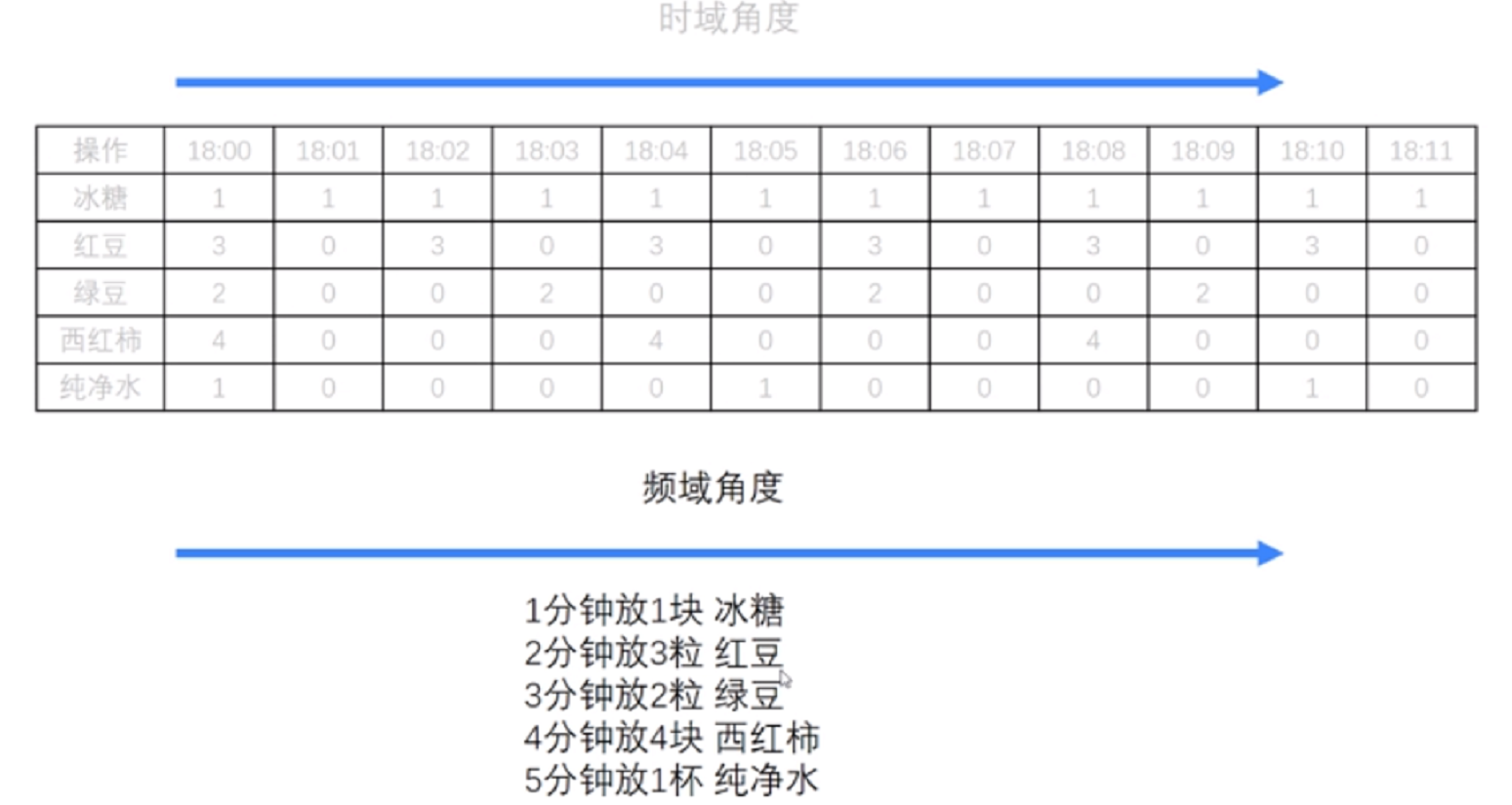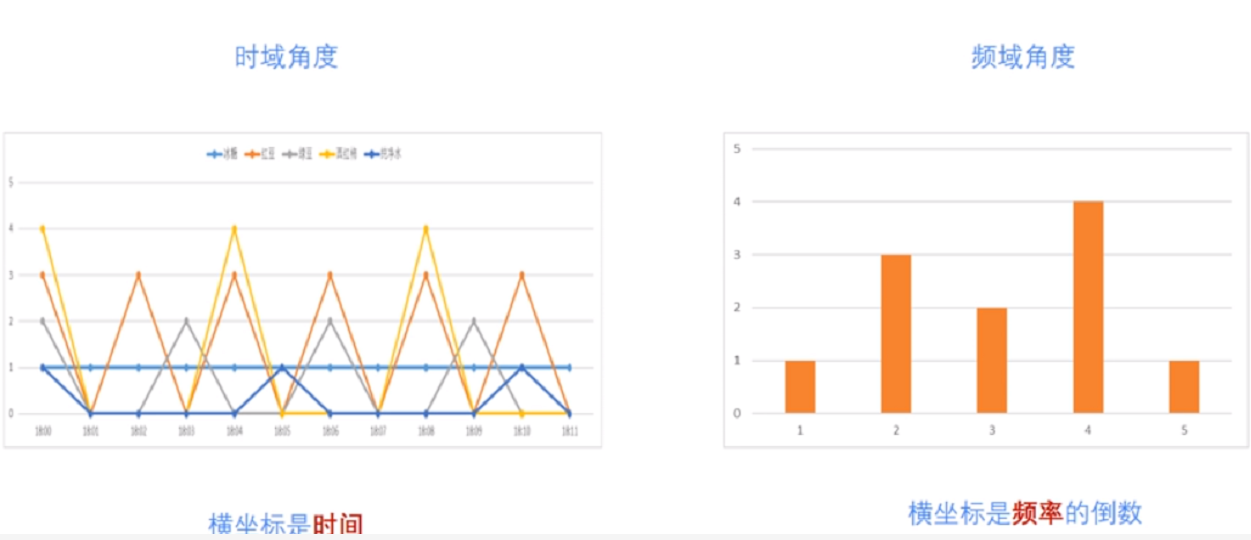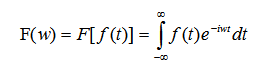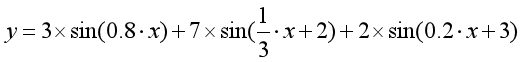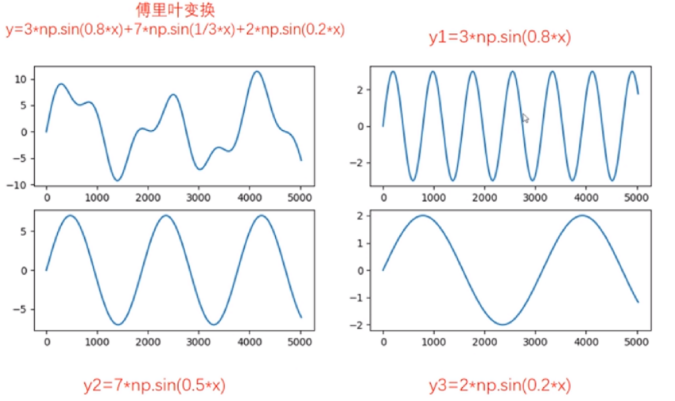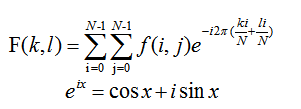# 二.Numpy实现傅里叶变换

Numpy中的 FFT包提供了函数 np.fft.fft2()可以对信号进行快速傅里叶变换，其函数原型如下所示，该输出结果是一个复数数组（Complex Ndarry）。

fft2(a, s=None, axes=(-2, -1), norm=None)

• a表示输入图像，阵列状的复杂数组
• s表示整数序列，可以决定输出数组的大小。输出可选形状（每个转换轴的长度），其中s表示轴0，s表示轴1。对应fit(x,n)函数中的n，沿着每个轴，如果给定的形状小于输入形状，则将剪切输入。如果大于则输入将用零填充。如果未给定’s’，则使用沿’axles’指定的轴的输入形状
• axes表示整数序列，用于计算FFT的可选轴。如果未给出，则使用最后两个轴。“axes”中的重复索引表示对该轴执行多次转换，一个元素序列意味着执行一维FFT
• norm包括None和ortho两个选项，规范化模式（请参见numpy.fft）。默认值为无

Numpy中的fft模块有很多函数，相关函数如下：

#计算一维傅里叶变换
numpy.fft.fft(a, n=None, axis=-1, norm=None)
#计算二维的傅里叶变换
numpy.fft.fft2(a, n=None, axis=-1, norm=None)
#计算n维的傅里叶变换
numpy.fft.fftn()
#计算n维实数的傅里叶变换
numpy.fft.rfftn()
#返回傅里叶变换的采样频率
numpy.fft.fftfreq()
#将FFT输出中的直流分量移动到频谱中央
numpy.fft.shift()

# -*- coding: utf-8 -*-
import cv2 as cv
import numpy as np
from matplotlib import pyplot as plt

#读取图像

#快速傅里叶变换算法得到频率分布
f = np.fft.fft2(img)

#默认结果中心点位置是在左上角,
#调用fftshift()函数转移到中间位置
fshift = np.fft.fftshift(f)

#fft结果是复数, 其绝对值结果是振幅
fimg = np.log(np.abs(fshift))

#展示结果
plt.subplot(121), plt.imshow(img, 'gray'), plt.title('Original Fourier')
plt.axis('off')
plt.subplot(122), plt.imshow(fimg, 'gray'), plt.title('Fourier Fourier')
plt.axis('off')
plt.show()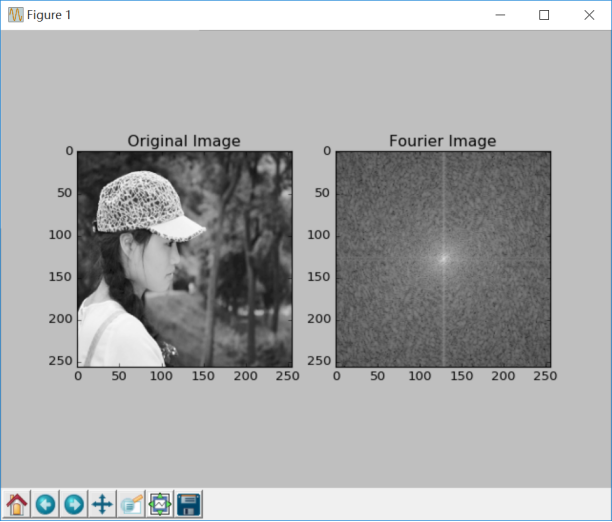# 三.Numpy实现傅里叶逆变换

#实现图像逆傅里叶变换，返回一个复数数组
numpy.fft.ifft2(a, n=None, axis=-1, norm=None)
#fftshit()函数的逆函数，它将频谱图像的中心低频部分移动至左上角
numpy.fft.fftshift()
#将复数转换为0至255范围
iimg = numpy.abs(逆傅里叶变换结果)

# -*- coding: utf-8 -*-
import cv2 as cv
import numpy as np
from matplotlib import pyplot as plt

#读取图像

#傅里叶变换
f = np.fft.fft2(img)
fshift = np.fft.fftshift(f)
res = np.log(np.abs(fshift))

#傅里叶逆变换
ishift = np.fft.ifftshift(fshift)
iimg = np.fft.ifft2(ishift)
iimg = np.abs(iimg)

#展示结果
plt.subplot(131), plt.imshow(img, 'gray'), plt.title('Original Image')
plt.axis('off')
plt.subplot(132), plt.imshow(res, 'gray'), plt.title('Fourier Image')
plt.axis('off')
plt.subplot(133), plt.imshow(iimg, 'gray'), plt.title('Inverse Fourier Image')
plt.axis('off')
plt.show()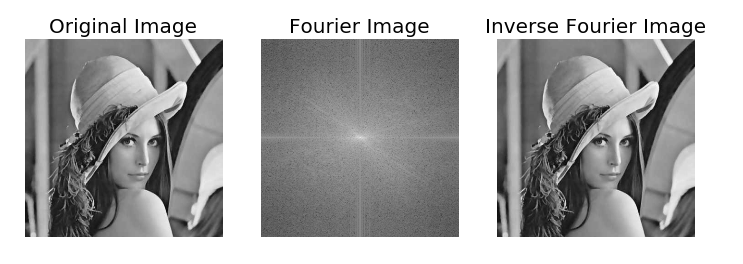# 四.OpenCV实现傅里叶变换

OpenCV 中相应的函数是cv2.dft()和用Numpy输出的结果一样，但是是双通道的。第一个通道是结果的实数部分，第二个通道是结果的虚数部分，并且输入图像要首先转换成 np.float32 格式。其函数原型如下所示：

dst = cv2.dft(src, dst=None, flags=None, nonzeroRows=None)

• src表示输入图像，需要通过np.float32转换格式
• dst表示输出图像，包括输出大小和尺寸
• flags表示转换标记，其中DFT _INVERSE执行反向一维或二维转换，而不是默认的正向转换；DFT _SCALE表示缩放结果，由阵列元素的数量除以它；DFT _ROWS执行正向或反向变换输入矩阵的每个单独的行，该标志可以同时转换多个矢量，并可用于减少开销以执行3D和更高维度的转换等；DFT _COMPLEX_OUTPUT执行1D或2D实数组的正向转换，这是最快的选择，默认功能；DFT _REAL_OUTPUT执行一维或二维复数阵列的逆变换，结果通常是相同大小的复数数组，但如果输入数组具有共轭复数对称性，则输出为真实数组
• nonzeroRows表示当参数不为零时，函数假定只有nonzeroRows输入数组的第一行（未设置）或者只有输出数组的第一个（设置）包含非零，因此函数可以处理其余的行更有效率，并节省一些时间；这种技术对计算阵列互相关或使用DFT卷积非常有用

cv2.magnitude(x, y)

• x表示浮点型X坐标值，即实部
• y表示浮点型Y坐标值，即虚部
最终输出结果为幅值，即：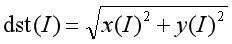# -*- coding: utf-8 -*-
import numpy as np
import cv2
from matplotlib import pyplot as plt

#读取图像

#傅里叶变换
dft = cv2.dft(np.float32(img), flags = cv2.DFT_COMPLEX_OUTPUT)

#将频谱低频从左上角移动至中心位置
dft_shift = np.fft.fftshift(dft)

#频谱图像双通道复数转换为0-255区间
result = 20*np.log(cv2.magnitude(dft_shift[:,:,0], dft_shift[:,:,1]))

#显示图像
plt.subplot(121), plt.imshow(img, cmap = 'gray')
plt.title('Input Image'), plt.xticks([]), plt.yticks([])
plt.subplot(122), plt.imshow(result, cmap = 'gray')
plt.title('Magnitude Spectrum'), plt.xticks([]), plt.yticks([])
plt.show()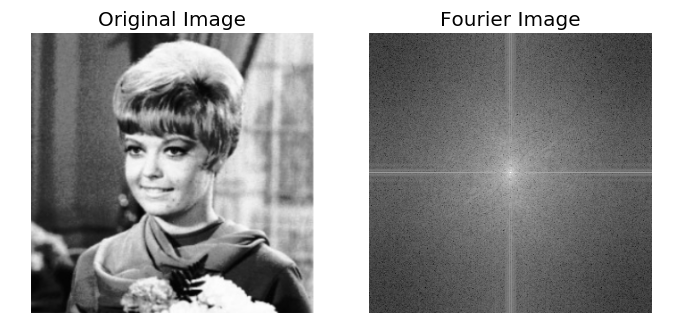# 五.OpenCV实现傅里叶逆变换

dst = cv2.idft(src[, dst[, flags[, nonzeroRows]]])

• src表示输入图像，包括实数或复数
• dst表示输出图像
• flags表示转换标记
• nonzeroRows表示要处理的dst行数，其余行的内容未定义（请参阅dft描述中的卷积示例）

# -*- coding: utf-8 -*-
import numpy as np
import cv2
from matplotlib import pyplot as plt

#读取图像

#傅里叶变换
dft = cv2.dft(np.float32(img), flags = cv2.DFT_COMPLEX_OUTPUT)
dftshift = np.fft.fftshift(dft)
res1= 20*np.log(cv2.magnitude(dftshift[:,:,0], dftshift[:,:,1]))

#傅里叶逆变换
ishift = np.fft.ifftshift(dftshift)
iimg = cv2.idft(ishift)
res2 = cv2.magnitude(iimg[:,:,0], iimg[:,:,1])

#显示图像
plt.subplot(131), plt.imshow(img, 'gray'), plt.title('Original Image')
plt.axis('off')
plt.subplot(132), plt.imshow(res1, 'gray'), plt.title('Fourier Image')
plt.axis('off')
plt.subplot(133), plt.imshow(res2, 'gray'), plt.title('Inverse Fourier Image')
plt.axis('off')
plt.show()# 六.总结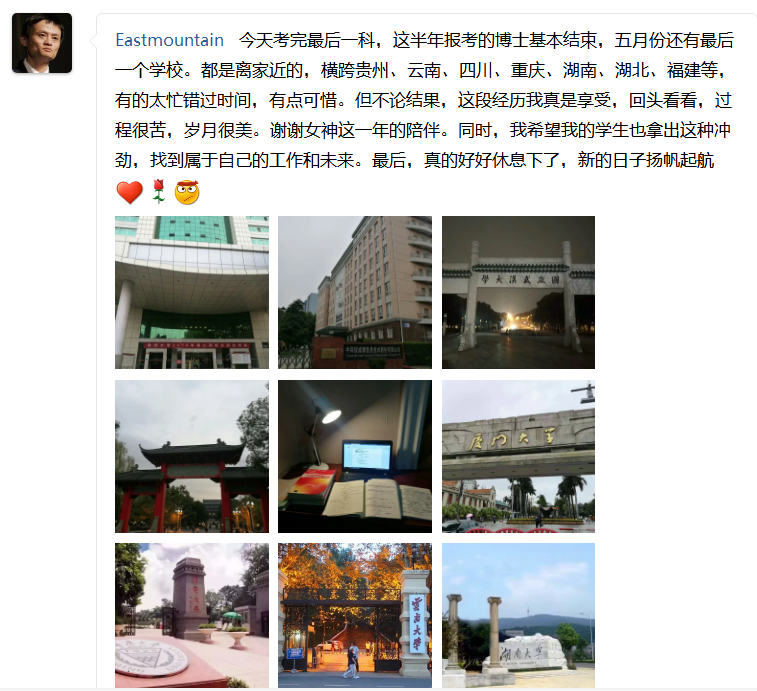（By：Eastmount 2019-04-23 周二下午6点写于花溪 https://blog.csdn.net/Eastmount )

## 图像处理之图像加噪

2012-01-06 15:53:38 jia20003 阅读数 27145
• ###### 再论图像分割

掌握图像去噪滤波方法及各自特点； 掌握常用的图像边缘检测算子，及Canny算子基本原理 掌握灰度阈值化及大津算法； 掌握常见的图像特征描述方式 了解局部阈值分割、区域生长、分水岭算法及基于轮廓的阈值分割； 通过...

2537人学习 CSDN就业班
免费试看

1.     椒盐噪声（Salt And Pepper Noise）

1. 指定信噪比 SNR 其取值范围在[0, 1]之间
2. 计算总像素数目 SP， 得到要加噪的像素数目 NP = SP * (1-SNR)
3. 随机获取要加噪的每个像素位置P（i, j）
4. 指定像素值为255或者0。
5. 重复c, d两个步骤完成所有像素的NP个像素
6. 输出加噪以后的图像

2.     高斯噪声（Gaussian Noise）

Pout = Pin + XMeans + sigma *G(d)

a.      输入参数sigam 和 X mean

b.      以系统时间为种子产生一个伪随机数

c.      将伪随机数带入G（d）得到高斯随机数

d.      根据输入像素计算出输出像素

e.      重新将像素值防缩在[0 ~ 255]之间

f.       循环所有像素

g.      输出图像

1.     椒盐噪声

int size= (int)(inPixels.length * (1-SNR));

for(int i=0; i<size; i++) {

int row = (int)(Math.random()* (double)height);

int col = (int)(Math.random()* (double)width);

index= row * width + col;

inPixels[index]= (255 << 24) | (255 << 16) | (255 << 8) | 255;

}

2.     高斯噪声

float d = (float)Math.random()*RANDOM_SCOPE - RANDOM_SCOPE/2;

float sigma2 = sigma*sigma*2;

float PI2 = (float)Math.PI * 2;

float sigmaPI2 = (float)Math.sqrt(PI2*sigma);

float result = (float)Math.exp(-d/sigma2)/sigmaPI2;

tr = (int)((float)tr + getGaussianValue() + this.means);

tg = (int)((float)tg + getGaussianValue() + this.means);

tb = (int)((float)tb + getGaussianValue() + this.means);

mean是的值为0.private BufferedImage addSaltAndPepperNoise(BufferedImage src, BufferedImage dst) {
int width = src.getWidth();
int height = src.getHeight();

if ( dst == null )
dst = createCompatibleDestImage( src, null );

int[] inPixels = new int[width*height];
getRGB( src, 0, 0, width, height, inPixels );

int index = 0;
int size = (int)(inPixels.length * (1-SNR));

for(int i=0; i<size; i++) {
int row = (int)(Math.random() * (double)height);
int col = (int)(Math.random() * (double)width);
index = row * width + col;
inPixels[index] = (255 << 24) | (255 << 16) | (255 << 8) | 255;
}

setRGB( dst, 0, 0, width, height, inPixels );
return dst;
}


private BufferedImage gaussianNoise(BufferedImage src, BufferedImage dst) {
int width = src.getWidth();
int height = src.getHeight();

if ( dst == null )
dst = createCompatibleDestImage( src, null );

int[] inPixels = new int[width*height];
int[][][] tempPixels = new int[height][width];
int[] outPixels = new int[width*height];
getRGB( src, 0, 0, width, height, inPixels );
int index = 0;
float inMax = 0;
float outMax = 0;
for(int row=0; row<height; row++) {
int ta = 0, tr = 0, tg = 0, tb = 0;
for(int col=0; col<width; col++) {
index = row * width + col;
ta = (inPixels[index] >> 24) & 0xff;
tr = (inPixels[index] >> 16) & 0xff;
tg = (inPixels[index] >> 8) & 0xff;
tb = inPixels[index] & 0xff;
if(inMax < tr) {
inMax = tr;
}
if(inMax < tg) {
inMax = tg;
}
if(inMax < tb) {
inMax = tb;
}
tr = (int)((float)tr + getGaussianValue() + this.means);
tg = (int)((float)tg + getGaussianValue() + this.means);
tb = (int)((float)tb + getGaussianValue() + this.means);
if(outMax < tr) {
outMax = tr;
}
if(outMax < tg) {
outMax = tg;
}
if(outMax < tb) {
outMax = tb;
}
tempPixels[row][col] = ta;
tempPixels[row][col] = tr;
tempPixels[row][col] = tg;
tempPixels[row][col] = tb;
}
}

// Normalization
index = 0;
float rate = inMax/outMax;
for(int row=0; row<height; row++) {
int ta = 0, tr = 0, tg = 0, tb = 0;
for(int col=0; col<width; col++) {
index = row * width + col;
ta = tempPixels[row][col];
tr = tempPixels[row][col];
tg = tempPixels[row][col];
tb = tempPixels[row][col];

tr = (int)((float)tr * rate);
tg = (int)((float)tg * rate);
tb = (int)((float)tb * rate);
outPixels[index] = (ta << 24) | (tr << 16) | (tg << 8) | tb;
}
}
setRGB( dst, 0, 0, width, height, outPixels );
return dst;
}